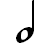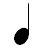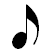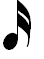Rhythm allows a musician to know how long a note should be played compared to other notes. Notes can be twice as long, half as long, 4 times as long, etc. as other notes. This relationship between rhythmic notes never changes.

## Basic Rhythmic Notes

The following are the most basic rhythmic notes:

 Whole NoteHalf NoteQuarter NoteEighth NoteSixteenth Note## Relating Rhythmic Notes

The names of rhythmic notes describe how the notes relate to each other, or fit together. Specifically, each note describes how many of itself fits into a whole note.

• A whole note is the base unit, so 1 whole note fits into a whole note.
• A half note is 1/2 the length of a whole note, so 2 half notes fit into a whole note.
• A quarter note is 1/4 (or one quarter, think of the coin compared to a dollar) the length of a whole note, so 4 quarter notes fit into a whole note.
• An eighth note is 1/8 the length of a whole note, so 8 eighth notes fit into a whole note.
• A sixteenth note is 1/16 the length of a whole note, so 16 sixteenth notes fit into a whole note.

The way in which these rhythmic notes fit together is illustrated on the chart below. Notice that the relationships described above for each note compared to the whole note can be seen below.

Also notice relationships between smaller notes. Here are some examples:

• 2 quarter notes take the same space as 1 half note.
• 4 sixteenth notes take the same space as 1 quarter note.
• 4 eighth notes take the same space as 1 half note.
• 8 sixteenth notes take the same space as 1 half note.
• 2 sixteenth notes take the same space as 1 eighth note.

These relationships or proportions regarding how rhythmic notes compare to each other never change. A half note will always be twice as long as a quarter note and half as long as a whole note.

Notice that we have not yet talked about how many beats each rhythmic note gets. That is because the number of beats that a note receives changes depending on the time signature. However, the rhythmic notes always fit together the same way.